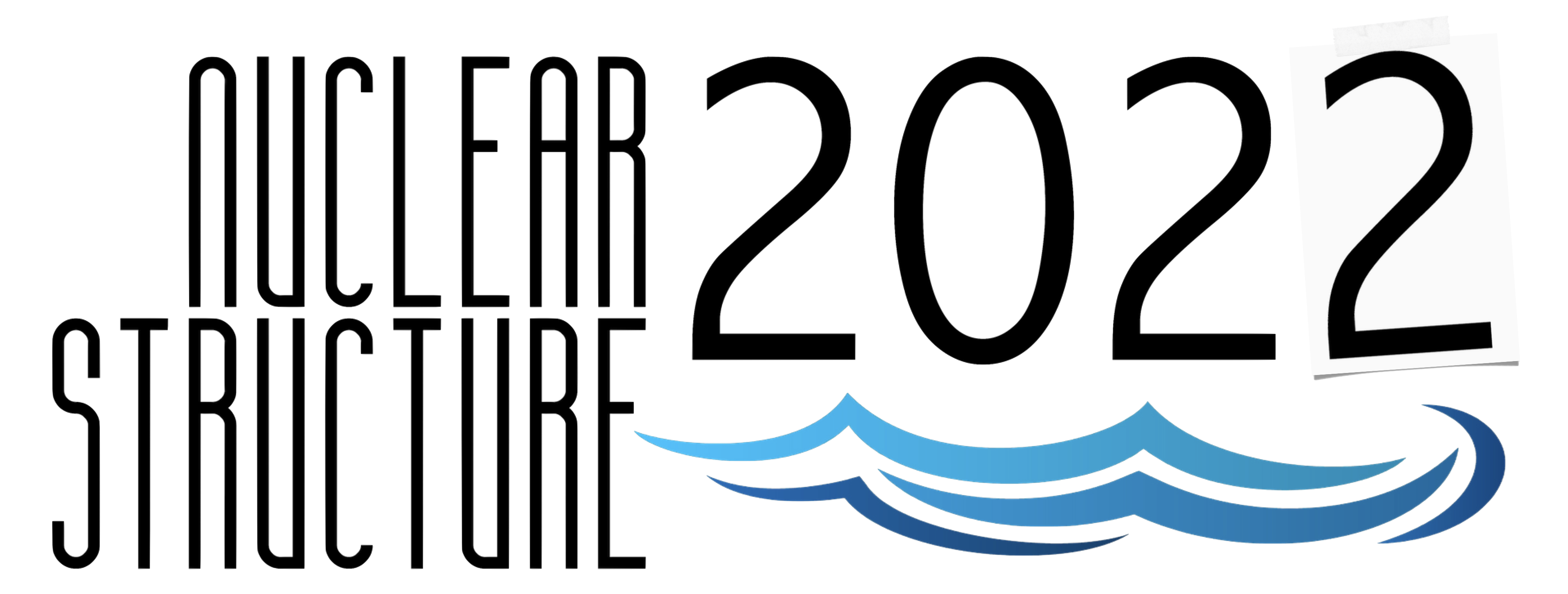#Nuclear Structure 2022

Jun 13 – 17, 2022
Berkeley, CA
US/Pacific timezone

## First Evidence of Axial Shape Asymmetry and Shape Coexistence in 74Zn: Suggestion for a Northern Extension of the N=40 Island of Inversion

Jun 14, 2022, 1:50 PM
20m
Berkeley, CA

#### Berkeley, CA

Lawrence Berkeley National Laboratory
Oral Oral Presentations

### Speaker

Marco Rocchini (Department of Physics, University of Guelph, N1G 2W1 Guelph, Canada)

### Description

Results from recent experiments studying nuclei in the $^{78}$Ni region suggest that the $N=50$ shell closure persists, in agreement with state-of-the-art shell-model calculations. However, how collectivity manifests and evolves in this region of the Segrè chart is still an open question, particularly concerning phenomena such as vibrational modes, axial shape asymmetry (triaxiality) and shape coexistence. This is especially true in the Zn isotopic chain in the neutron-rich region, in which even definitive spin assignments are unavailable except for the very low-lying states.
In this talk, I will present the results of a recent experiment performed at the TRIUMF laboratory (Vancouver, Canada) using the GRIFFIN $\gamma$-ray spectrometer. The excited states of $^{74}$Zn were investigated via $\gamma$-ray spectroscopy following $^{74}$Cu $\beta$-decay. By exploiting $\gamma$-$\gamma$ angular correlation analysis, the $2_2^+$, $3_1^+$, $0_2^+$ and $2_3^+$ states in $^{74}$Zn were firmly identified. The $\gamma$-ray branching and $E2/M1$ mixing ratios for transitions de-exciting the $2_2^+$, $3_1^+$ and $2_3^+$ states were measured, allowing for the extraction of relative $B(E2)$ values. In particular, the $2_3^+ \to 0_2^+$ and $2_3^+ \to 4_1^+$ transitions were observed for the first time. The levels observed were organized into rotational-like bands and the results were compared with large-scale shell-model calculations from which the shapes of individual states were determined. Enhanced triaxiality is suggested to characterize $^{74}$Zn in its ground state. Furthermore, an excited $K=0$ band with a different shape is identified. A shore of the $N=40$ island of inversion appears to manifest above $Z=28$, previously thought as its northern limit in the nuclide chart.